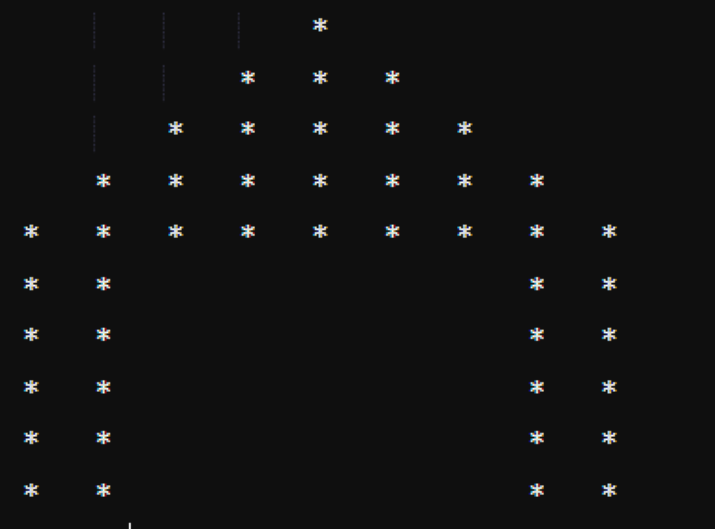# Program to print Hut Star pattern

Solving interesting pattern problems enhances the understanding of loops. They are essential because they help in building a strong foundation of a particular programming language. There are various kinds of patterns including number-based, star-based and alphabetical patterns as well. This article will guide you to solve a hut star pattern using nested for loop in Java.

## Java Program to print Hut Star Pattern

Since we are going to solve the problem using nested for loop therefore, it is necessary to discuss its syntax.

### Syntax

for ( initial expression; conditional expression; increment/decrement expression ){
for ( initial expression; conditional expression; increment/decrement expression ) {
// code to be executed
}
}


initial expression − executed once when loop begins.

conditional expression − code will be executed till conditional expression is true.

increment/decrement expression − to increment/decrement loop variable.

### Pattern### Approach

• Divide the whole pattern into two parts. First as an upper triangular shape and the second as a lower rectangular part.

• Declare and initialize an integer ‘n’ that specifies number of rows for upper and lower part.

• Declare and initialize initial count of space and stars.

• Now, for the upper triangular part define a nested for loop. The outer for loop will run till ‘n’ and the first inner loop will run till space count and print the spaces. After printing decrement the space count by 1.

• The second inner for loop will run till star count and print the stars. After printing increment the star count by 2.

• Again create another nested for loop. The outer for loop will run till ‘n’ and the first inner loop will print the left rectangular shape, the second inner loop will print the spaces and last inner loop will print right rectangular shape.

### Example

public class Hut {
public static void main(String[] args) {
// count of upper triangle row and lower rectangle row
int n = 5;
int spc = n-1;
// initial count of space
int str = 1;
// initial count of star
// upper triangular shape
for(int i = 1; i <= n; i++) {
for(int j = 1; j <= spc; j++) {
// for space
System.out.print("\t");
}
spc--;
for(int k = 1; k <= str; k++) {
// for star
System.out.print("*\t");
}
str += 2;
System.out.println();
// to move the cursor to next line
}
// lower rectangular shape
for (int i = 0; i < n; i++) {
// for left rectangular shape
for (int j = 0; j < n/2; j++) {
System.out.print("*\t");
}
// for space
for (int j = 0; j < 5; j++) {
System.out.print("\t");
}
// for right rectangular shape
for (int j = 0; j < n/2; j++) {
System.out.print("*\t");
}
System.out.println();
// to move the cursor to next line
}
}
}


### Output

				*
*	*	*
*	*	*	*	*
*	*	*	*	*	*	*
*	*	*	*	*	*	*	*	*
*	*						*	*
*	*						*	*
*	*						*	*
*	*						*	*
*	*						*	*


## Conclusion

In this article, we have discussed the solution of the hut star pattern. We solved this particular problem with the help of nested for loop. This will help you to decode the logic of the pattern questions and make you capable of solving other patterns on your own.

Updated on: 16-May-2023

346 Views